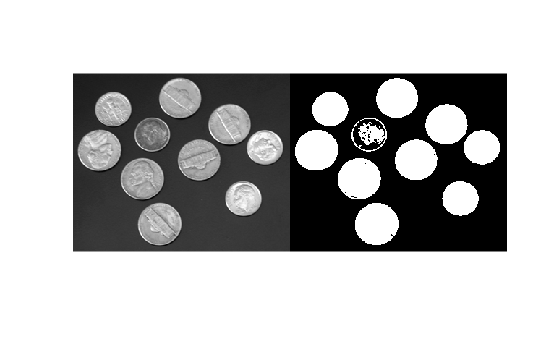# graythresh

Global image threshold using Otsu's method

## Syntax

``T = graythresh(I)``
``````[T,EM] = graythresh(I)``````

## Description

example

````T = graythresh(I)` computes a global threshold `T` from grayscale image `I`, using Otsu's method . Otsu's method chooses a threshold that minimizes the intraclass variance of the thresholded black and white pixels. The global threshold `T` can be used with `imbinarize` to convert a grayscale image to a binary image.```
``````[T,EM] = graythresh(I)``` also returns the effectiveness metric, `EM`.```

## Examples

collapse all

Read a grayscale image into the workspace.

`I = imread('coins.png');`

Calculate a threshold using `graythresh`. The threshold is normalized to the range [0, 1].

`level = graythresh(I)`
```level = 0.4941 ```

Convert the image into a binary image using the threshold.

`BW = imbinarize(I,level);`

Display the original image next to the binary image.

`imshowpair(I,BW,'montage')`## Input Arguments

collapse all

Grayscale image, specified as a numeric array of any dimensionality. The `graythresh` function converts multidimensional arrays to 2-D arrays using the `reshape` function, and ignores any nonzero imaginary part of `I`.

Data Types: `single` | `double` | `int16` | `uint8` | `uint16`

## Output Arguments

collapse all

Global threshold, returned as a nonnegative number in the range [0, 1].

Data Types: `double`

Effectiveness metric of the threshold, returned as a nonnegative number in the range [0, 1]. The lower bound is attainable only by images having a single gray level, and the upper bound is attainable only by two-valued images.

Data Types: `double`

## Tips

• By default, the function `imbinarize` creates a binary image using a threshold obtained using Otsu’s method. This default threshold is identical to the threshold returned by `graythresh`. However, `imbinarize` only returns the binary image. If you want to know the level or the effectiveness metric, use `graythresh` before calling `imbinarize`.

 Otsu, N., "A Threshold Selection Method from Gray-Level Histograms." IEEE Transactions on Systems, Man, and Cybernetics. Vol. 9, No. 1, 1979, pp. 62–66.